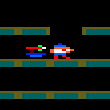### CPC Basic

Would you like to react to this message? Create an account in a few clicks or log in to continue.

# Greetings / Vertical Stars moving across the Screen.Out Bush

Posts : 11
Join date : 2019-12-02
Hello,

I too was trying my hand at coding in CPC BASIC 3 when it was initially released, though fell into the trap of thinking along the lines of Locomotive BASIC when CPC BASIC 3 is a little bit different.

Recently I have created some CPC BASIC stuff which I'll post in the coming week. Back in 2016 I started creating a game which I ended up finishing with Hisoft Pascal called Get The Cash, however I've gone back to that early version, unfortunately I can't remember what the problem was with it when I originally switched languages, I have a feeling it was to do with the Graphics moving down the screen, but I'll get to in due course.

What I have now is some Vertical Stars I started in Locomotive BASIC, though a few years ago I tried converting to CPC BASIC for performance, though there were problems. A few days ago I had a look and realised that Stars were being Plotted Any old spot on the Graphics screen, so when they would move by being subtracted by 4 on line 250, it would reach a point many of those would go below 0. The solution was to make sure each Star pixel would be placed into a spot divisible by 4, which I did in the following code below by forcing a WHILE loop in 150 to 170 to only exit when a number divisible by 4 is selected.

Code:
`100 DEFWORD a-z:DIM a(20)110 FOR n=1 TO 20 STEP 2120  x=n130  y=n+1140  v=RND MOD 636+4150  WHILE v MOD 4 <> 0160    v=RND MOD 636+4170  WEND180  a(x)=v190  a(y)=RND MOD 398200 NEXT n210 MODE 0:INK 0,0:INK 1,26220 WHILE 1230  FOR n=1 TO 20 STEP 2240  x=n:y=n+1250  IF a(x)>0 THEN PLOT a(x),a(y),0:a(x)=a(x)-4:PLOT a(x),a(y),1260  IF a(x)=0 THEN PLOT a(x),a(y),0:GOSUB 290:PLOT a(x),a(y),1270 NEXT n280 WEND290 a(x)=640300 a(y)=RND MOD 398310 RETURN`

Current date/time is Thu Aug 18, 2022 7:25 pm